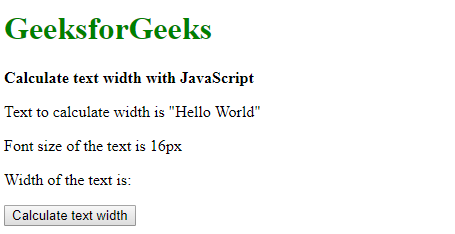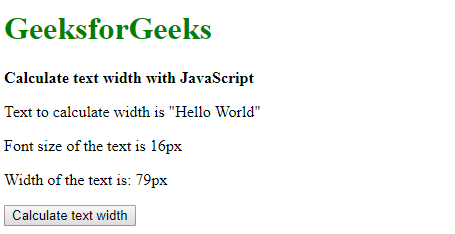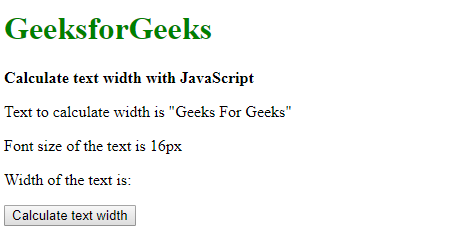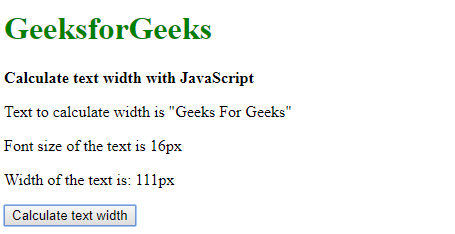# Calculate the width of the text in JavaScript

Method 1: Creating a new DOM Element and measuring its width: A new “span” element is created with the createElement() method. Then added it to the body of the element using the appendChild() method. The style property of this element is used to set properties like the font, font-size, height, width, whiteSpace, and position.
The font is set to the string with the font needed. The font-size is a string with a value in pixels. The height and width properties are set to ‘auto’. The position property is set to ‘absolute’ and the whiteSpace property is set to ‘no-wrap’. The text to be measured is specified using the innerHTML property. The clientWidth property is used to get the width of its element. This value will represent the width of text in pixels. The element is then removed using the removeChild() method.

Example:

 ` ` `<``html``> ` `     `  `<``head``> ` `    ``<``title``> ` `        ``Calculate the text width ` `        ``with JavaScript  ` `    `` ` ` ` ` `  `<``body``> ` `    ``<``h1` `style``=``"color: green"``> ` `        ``GeeksforGeeks ` `    `` ` `     `  `    ``<``b``> ` `        ``Calculate text width  ` `        ``with JavaScript ` `    ``  ` `     `  `    ``<``p``> ` `        ``Text to calculate width is ` `        ``"Hello World" ` `    `` ` `    ``<``p``>Font size of the text is 16px ` `     `  `    ``<``p``> ` `        ``Width of the text is: ` `        ``<``span` `class``=``"output"``> ` `    `` ` `     `  `    ``<``button` `onclick``=``"getTextWidth()"``> ` `        ``Calculate text width ` `    `` ` `     `  `    ``<``script` `type``=``"text/javascript"``> ` `        ``function getTextWidth() { ` ` `  `            ``text = document.createElement("span"); ` `            ``document.body.appendChild(text); ` ` `  `            ``text.style.font = "times new roman"; ` `            ``text.style.fontSize = 16 + "px"; ` `            ``text.style.height = 'auto'; ` `            ``text.style.width = 'auto'; ` `            ``text.style.position = 'absolute'; ` `            ``text.style.whiteSpace = 'no-wrap'; ` `            ``text.innerHTML = 'Hello World'; ` ` `  `            ``width = Math.ceil(text.clientWidth); ` `            ``formattedWidth = width + "px"; ` ` `  `            ``document.querySelector('.output').textContent ` `                    ``= formattedWidth; ` `            ``document.body.removeChild(text); ` `        ``} ` `    `` ` ` ` ` `  `     `

Output:

• Before clicking the button:• After clicking the button:Method 2: Using the canvas measureText() method: A new “canvas” element is created with the createElement() method. The 2d context required to draw graphics is accessed using the getContext() method and passing the value “2d” as the parameter. This context will be used to manipulate the text in the canvas. The font is specified using the font property. The font string uses the same syntax as a CSS font specifier.
The dimensions of the text are calculated using the measureText() method. The text to be measured is passed to this method. It returns a TextMetrics object that contains information about the measured text. The width property of this TextMetrics object is used to get the width of the text. The width may be a floating-point value, hence the Math.ceil() function is used to find the ceiling of the floating-point and return an integer. This value is the width of the measured text in pixels.

Example:

 ` ` `<``html``> ` ` `  `<``head``> ` `    ``<``title``> ` `        ``Calculate the text width with JavaScript ` `    `` ` ` ` ` `  `<``body``> ` `    ``<``h1` `style``=``"color: green"``> ` `        ``GeeksforGeeks ` `    `` ` `     `  `    ``<``b``>Calculate text width with JavaScript  ` `     `  `    ``<``p``> ` `        ``Text to calculate width is  ` `        ``"Geeks For Geeks" ` `    `` ` `     `  `    ``<``p``>Font size of the text is 16px ` `     `  `    ``<``p``> ` `        ``Width of the text is:  ` `        ``<``span` `class``=``"output"``> ` `    `` ` `     `  `    ``<``button` `onclick``=``"getTextWidth()"``> ` `        ``Calculate text width ` `    `` ` `     `  `    ``<``script` `type``=``"text/javascript"``> ` `        ``function getTextWidth() { ` ` `  `            ``inputText = "Geeks For Geeks"; ` `            ``font = "16px times new roman"; ` ` `  `            ``canvas = document.createElement("canvas"); ` `            ``context = canvas.getContext("2d"); ` `            ``context.font = font; ` `            ``width = context.measureText(inputText).width; ` `            ``formattedWidth = Math.ceil(width) + "px"; ` ` `  `            ``document.querySelector('.output').textContent ` `                        ``= formattedWidth; ` `        ``} ` `    `` ` ` ` ` `  `     `

Output:

• Before clicking the button:• After clicking the button:My Personal Notes arrow_drop_upCheck out this Author's contributed articles.

If you like GeeksforGeeks and would like to contribute, you can also write an article using contribute.geeksforgeeks.org or mail your article to contribute@geeksforgeeks.org. See your article appearing on the GeeksforGeeks main page and help other Geeks.

Please Improve this article if you find anything incorrect by clicking on the "Improve Article" button below.